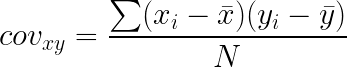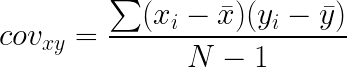# Covariance

Or input your data as csv

Sharing helps us build more free tools

Quickly calculate covariance with this free online covariance calculator.

Covariance measures how much two variables, x and y, vary together. It is similar to correlation but the result does not get normalized to be between -1 and 1.

If x and y have a positive covariance, then when x is large y is also large. When x is small then y is also small.

If x and y have a very negative covariance, then when x is large y is small. When x is small then y is large.

If x and y have zero covariance, then changes in x do not correlate with changes in y. When x increases y does not always increase or decrease.

## When To Use Covariance

Covariance is useful when you want to figure out if two variables change together. If two variables change together, then it might be possible to change one by changing the other.

If two variables have a covariance close to 0, then they are not likely related.

Regression can also be used to quantify the relationship between variables.

## How To Calculate Covariance

2. ### View the covariances between columns in your dataset

See what columns have the highest and lowest covariance

## Covariance Equation

The covariance formula depends on whether your data set contains the whole population or only a sample.

If the number of data values is high, then the sample and population covariances will be very similar.

## Population Covariance Formula

The covariance of a sample can be calculated using the following formula.x_i is the value of x. x bar is the mean of x values.

y_i is the value of y. y bar is the mean of all y values.

N is the number of data values

## Sample Covariance Formula

The covariance of a sample can be calculated using the following formulax_i is the value of x. x bar is the mean of x values.

y_i is the value of y. y bar is the mean of all y values.

N is the number of data values

## Covariance Value vs Correlation Coefficient

The correlation coefficient can be calculated by dividing the covariance by the product of x and y standard deviations. This division normalizes the result to be between -1 and 1.

The correlation coefficient can be used to gauge the strength of the relationship between two variables because it is normalized to be between -1 and 1.